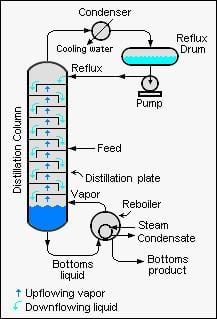# Fenske equation

Updated onThe Fenske equation in continuous fractional distillation is an equation used for calculating the minimum number of theoretical plates required for the separation of a binary feed stream by a fractionation column that is being operated at total reflux (i.e., which means that no overhead product distillate is being withdrawn from the column).

## Contents

The equation was derived in 1932 by Merrell Fenske, a professor who served as the head of the chemical engineering department at the Pennsylvania State University from 1959 to 1969.

When designing large-scale, continuous industrial distillation towers, it is very useful to first calculate the minimum number of theoretical plates required to obtain the desired overhead product composition.

## Common versions of the Fenske equation

This is one of the many different but equivalent versions of the Fenske equation valid only for binary mixtures:

N = log [ ( X d 1 X d ) ( 1 X b X b ) ] log α a v g

where:

• N is the minimum number of theoretical plates required at total reflux (of which the reboiler is one),
• X d  is the mole fraction of more volatile component in the overhead distillate,
• X b  is the mole fraction of more volatile component in the bottoms,
• α a v g  is the average relative volatility of the more volatile component to the less volatile component.
• For a multi-component mixture the following formula holds. For ease of expression, the more volatile and the less volatile components are commonly referred to as the light key (LK) and the heavy key (HK), respectively. Using that terminology, the above equation may be expressed as:

N = log [ ( L K d H K d ) ( H K b L K b ) ] log α a v g

or also:

N = log [ ( L K d 1 L K d ) ( 1 L K b L K b ) ] log α a v g

If the relative volatility of the light key to the heavy key is constant from the column top to the column bottom, then α a v g . is simply α . If the relative volatility is not constant from top to bottom of the column, then the following approximation may be used:

α a v g . = ( α t ) ( α b )

where:

• α t is the relative volatility of light key to heavy key at top of column,
• α b is the relative volatility of light key to heavy key at bottom of column.
• The above forms of the Fenske equation can be modified for use in the total reflux distillation of multi-component feeds. It is also helpful in solving liquid–liquid extraction problems, because an extraction system can also be represented as a series of equilibrium stages and relative solubility can be substituted for relative volatility.

## Another form of the Fenske equation

A derivation of another form of the Fenske equation for use in gas chromatography is available on the U.S. Naval Academy's web site. Using Raoult's law and Dalton's Law for a series of condensation and evaporation cycles (i.e., equilibrium stages), the following form of the Fenske equation is obtained:

Z a Z b = X a X b ( P a 0 P b 0 ) N

where:

• N  is the number of equilibrium stages,
• Z n  is the mole fraction of component n in the vapor phase,
• X n  is the mole fraction of component n in the liquid phase,
• P n 0  is the vapor pressure of pure component n.
• ## References

Similar Topics
Cop Land
Paulino Bernal
William Albert Norris
Topics